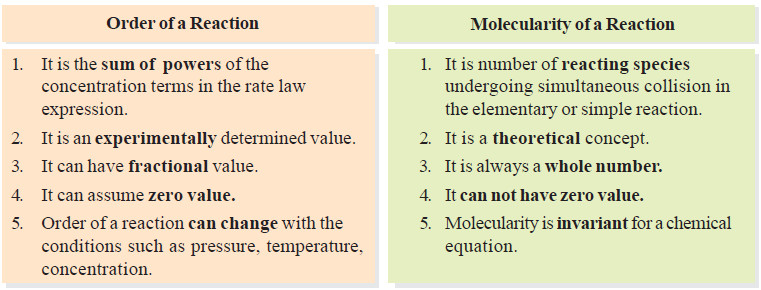# Molecularity of a reaction

– we will discuss the Molecularity of a reaction and the Differences Between Order and Molecularity.

## Molecularity of a reaction

– Chemical reactions may be classed into two types :

(a) Elementary reactions

(b) Complex reactions

– An elementary reaction is a simple reaction which occurs in a single step.

– A complex reaction is that which occurs in two or more steps.

## Molecularity of an Elementary Reaction

– The molecularity of an elementary reaction is defined as : the number of reactant molecules involved in a reaction.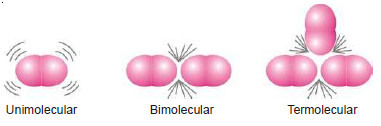– Thus the molecularity of an elementary reaction is 1, 2, 3, etc., according as one, two or three reactant molecules are participating in the reaction.

– The elementary reactions having molecularity 1, 2 and 3 are called unimolecular, bimolecular and termolecular respectively.

– Thus we have :

(a) Unimolecular reactions : (molecularity: 1)

(b) Bimolecular reactions : (molecularity = 2)

(c) Termolecular reactions : (molecularity = 3)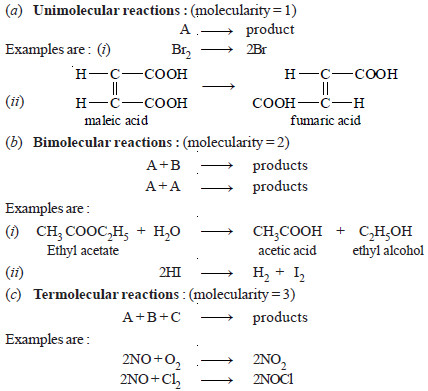## Why High Molecularity Reactions are Rare ?

– Most of the reactions involve one, two or at the most three molecules.

– The reactions involving four or more molecules are very rare.

– The rarity of reactions with high molecularity can be explained on the basis of the kinetic molecular theory.

– According to this theory, the rate of a chemical reaction is proportional to the number of collisions taking place between the reacting molecules.

– The chances of simultaneous collision of reacting molecules will go on decreasing with increase in number of molecules.

– Thus the possibility of three molecules colliding together is much less than in case of bimolecular collision.

– For a reaction of molecularity 4, the four molecules must come closed and collide with one another at the same time.

– The possibility of their doing so is much less than even in the case of termolecular reaction.

– Hence the reactions involving many molecules proceed through a series of steps, each involving two or three or less number of molecules.

– Such a reaction is called a complex reaction and the slowest step determines the overall rate of the reactions.

## Molecularity of a Complex Reaction

– Most chemical reactions are complex reactions.

– These occur in a series of steps. Each step is an elementary reaction.

– The stepwise sequence of elementary reactions that convert reactions to products is called the mechanism of the reaction.

– In any mechanism, some of the steps will be fast, others will be slow.

– A reaction can proceed no faster than its slowest step.

– Thus the slowest step is the ratedetermining step of the reaction.

– The decomposition of N2O5,

2N2O5 ⎯⎯→ 4NO2 + O2

is an example of a complex reaction. It occurs by the following steps :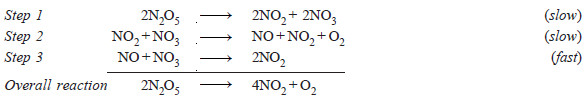– Each elementary reaction has its own molecularity equal to the number of molecules or atoms participating in it.

– It is meaningless to give the molecularity of the overall reaction because it is made of several elementary reactions, each, perhaps with a different molecularity.

– At best could be thought of as : the number of molecules or atoms taking part in the rate-determining step.

– Thus step 2 in the above mechanism is rate-determining and has molecularity ‘2’ which could be considered as the molecularity of the decomposition reaction of N2O5.

## Molecularity versus order of reaction

– The term molecularity is often confused with order of a reaction.

– The total number of molecules or atoms which take part in a reaction as represented by the chemical equation, is known as the molecularity of reaction.

– The sum of the powers to which the concentrations are raised in the rate law is known as the order of reaction.

### Molecularity and Order are Identical for Elementary Reactions or Steps

– The rate of an elementary reaction is proportional to the number of collisions between molecules (or atoms) of reactions.

– The number of collisions in turn is proportional to the concentration of each reactant molecule (or atom). Thus for a reaction.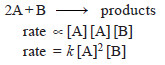– Two molecules of A and one molecule of B are participating in the reaction and, therefore, molecularity of the reaction is 2 + 1 = 3.

– The sum of powers in the rate law is 2 + 1 and hence the reaction order is also 3. Thus the molecularity and order for an elementary reaction are equal.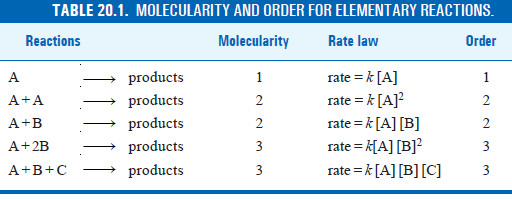## Differences Between Order and Molecularity